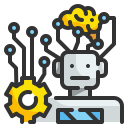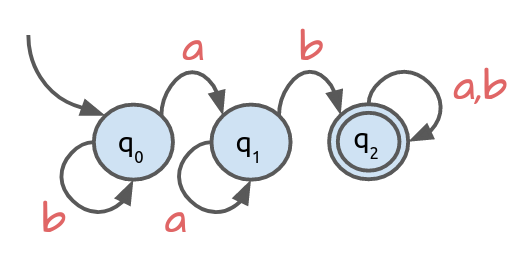# Problem Set #6: Automata

### Objective

During this activity, students should be able to:

• Design and implement simulations for deterministic finite automata (DFA).

This activity helps students develop the following skills, values and attitudes: ability to analyze and synthesize, capacity for identifying and solving problems, and efficient use of computer systems.

## Activity Description

Solve with your team the Clojure exercises described in this problem set. Make sure the code passes all the unit tests.

Create a namespace called automata. At the begining of the file add the following code in order to declare the namespace and import the required external functions:

(ns automata
(:require [clojure.test :refer [deftest is run-tests]]))


(run-tests)

All the code you write should go between these two instructions.

1. Implement a deterministic finite automata (DFA) simulator. In order to do this, write a function called accepts? that takes two arguments: $$\textit{dfa}$$ and $$\textit{input}$$, where $$\textit{dfa}$$ is an object that holds all the information associated to an automaton, and $$\textit{input}$$ is a string with a sequence of symbols (Clojure characters) that belong to the automaton’s alphabet. The function returns true if $$\textit{input}$$ gets accepted by $$\textit{dfa}$$, or false if gets rejected.

Test the function using the following state diagram:For the following unit tests, dfa-1 is assumed to be the binding to the corresponing automaton object.

(deftest test-problem1
(is (accepts? dfa-1 "ab"))
(is (accepts? dfa-1 "abba"))
(is (accepts? dfa-1 "aaab"))
(is (accepts? dfa-1 "abbbbbbbbb"))
(is (not (accepts? dfa-1 "")))
(is (not (accepts? dfa-1 "a")))
(is (not (accepts? dfa-1 "baa")))
(is (not (accepts? dfa-1 "bbba"))))

2. From the alphabet $$\Sigma = \{0, 1\}$$, design and code an automaton called dfa-2 that accepts strings that start with $$0$$ and end with $$1$$, and rejects all other strings.

Unit tests:

(deftest test-problem2
(is (accepts? dfa-2 "01"))
(is (accepts? dfa-2 "0101"))
(is (accepts? dfa-2 "01111"))
(is (accepts? dfa-2 "000001"))
(is (not (accepts? dfa-2 "")))
(is (not (accepts? dfa-2 "00")))
(is (not (accepts? dfa-2 "1001011")))
(is (not (accepts? dfa-2 "1001010"))))

3. Design and code an automaton called dfa-3 with alphabet $$\Sigma = \{x, y\}$$, that accepts any string that contains a sequence of three consecutive $$y$$ symbols, and rejects anything else.

Unit tests:

(deftest test-problem3
(is (accepts? dfa-3 "yyy"))
(is (accepts? dfa-3 "xyxyyyx"))
(is (accepts? dfa-3 "xxxxxyyyyy"))
(is (accepts? dfa-3 "yyyxxxxyyy"))
(is (not (accepts? dfa-3 "")))
(is (not (accepts? dfa-3 "xxx")))
(is (not (accepts? dfa-3 "yxxyxxy")))
(is (not (accepts? dfa-3 "xyxyyxyyx"))))

4. Design and code an automaton called dfa-4 with alphabet $$\Sigma = \{ i, j, k \}$$, that accepts strings that have an even length and rejects strings that have an odd length.

Unit tests:

(deftest test-problem4
(is (accepts? dfa-4 ""))
(is (accepts? dfa-4 "ji"))
(is (accepts? dfa-4 "iiiijjjjkkkk"))
(is (accepts? dfa-4 "kjikjikjikjikjikjikjikji"))
(is (not (accepts? dfa-4 "i")))
(is (not (accepts? dfa-4 "ijk")))
(is (not (accepts? dfa-4 "jjjjjiiiiikkkkk")))
(is (not (accepts? dfa-4
"kjikjikjikjikjikjikjikjikji"))))

5. From the alphabet $$\Sigma = \{ s, t \}$$, design and code an automaton called dfa-5 that rejects any string that contains a sequence of two or more repeated consecutive symbols and accepts anything else.

Unit tests:

(deftest test-problem5
(is (accepts? dfa-5 ""))
(is (accepts? dfa-5 "s"))
(is (accepts? dfa-5 "stststs"))
(is (accepts? dfa-5 "tststststststs"))
(is (not (accepts? dfa-5 "ss")))
(is (not (accepts? dfa-5 "ststststt")))
(is (not (accepts? dfa-5
"tstststsststststsssts")))
(is (not (accepts? dfa-5
"tttttttttttttttttttttttttt"))))

6. Design and code an automaton called dfa-6 with alphabet $$\Sigma = \{\#, \\}$$, that accepts strings where each $$\#$$ symbol is followed by exactly one or three $$\$$ symbols, and rejects anything else.

Unit tests:

(deftest test-problem6
(is (accepts? dfa-6 ""))
(is (accepts? dfa-6 "$$")) (is (accepts? dfa-6 "$$$#$#$$#")) (is (accepts? dfa-6 "#$$$#$#$$##$$$#$#$")) (is (not (accepts? dfa-6 "#"))) (is (not (accepts? dfa-6 "$$##$$#$$"))) (is (not (accepts? dfa-6 "$$$$####$$$$#"))) (is (not (accepts? dfa-6 "#####$$$#$$#$$\$#"))))

7. From the alphabet $$\Sigma = \{ @, \% \}$$, design and code an automaton called dfa-7 that accepts any string that contains exactly two not necessarily consecutive $$@$$ symbols and rejects any thing else.

Unit tests:

(deftest test-problem7
(is (accepts? dfa-7 "@@"))
(is (accepts? dfa-7 "%@%@%"))
(is (accepts? dfa-7 "@%%%%%%%%%@%%"))
(is (accepts? dfa-7 "%%%%%%@@%%%%%%%%%%"))
(is (not (accepts? dfa-7 "")))
(is (not (accepts? dfa-7 "%@%")))
(is (not (accepts? dfa-7 "@@@@@@@@@@@@")))
(is (not (accepts? dfa-7 "@%%%%@%%%%%@%%%"))))


## Deliverables

The program source file must include at the top the authors’ personal information (student ID and name) within comments. For example:

;----------------------------------------------------------
; Problem Set #6: Automata
; Date: April 28, 2023.
; Authors:
;          A01770771 Sylvie Laufeydottir
;          A01777771 Loki Laufeyson
;----------------------------------------------------------


Also, each function should include a documentation string (docstring) with a brief description of its behavior. For example:

(defn max2
"Returns the largest of the two numbers x and y."
[x y]
(if (> x y) x y))


### Instrucciones para subir archivo

Para entregar el archivo automata.clj, ingresa los siguientes datos:

Only one team member needs to upload the file.

Due date is Friday, April 28.

## Evaluation

This activity will be evaluated using the following criteria:

 -10 The program doesn't contain within comments the author’s personal information. A docstring is missing in one or more functions. The program contains syntax errors. The program was plagiarized in whole or in part. Depending on the amount of exercises that were solved correctly.